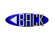# Algeria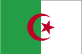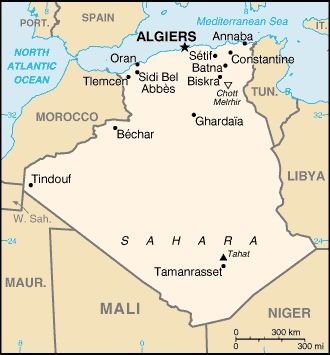# OVAL : DZ

Passenger series
Diplomatic series

## Passenger series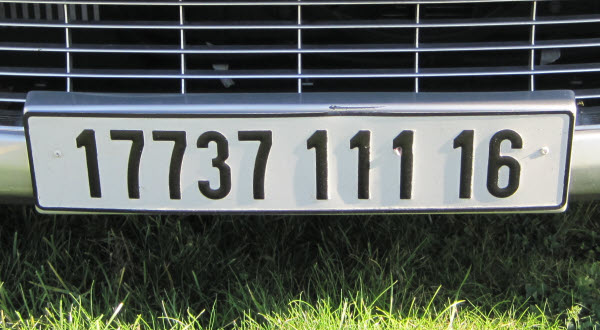Current passenger series since 1976, front plate in black on white.
The registration consists of five numerals, followed by three numerals and two numerals.
The first numeral of the second group denotes the vehicle class where 1 stands for private cars.
The second and third numeral of that second group denote the year of manufacture where 11 stands for 2011.
The last two numerals denote the wilaya (state) where 16 stands for El Djezair (Algiers).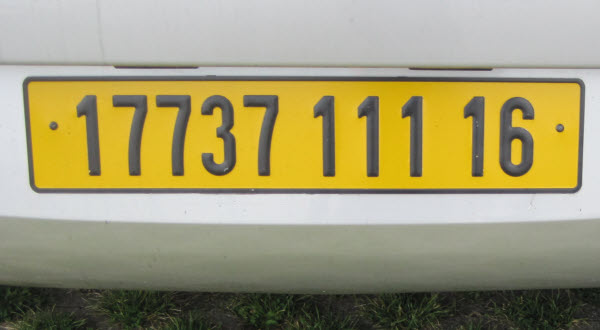Current passenger series since 1976, rear plate in black on yellow.
The registration consists of five numerals, followed by three numerals and two numerals.
The first numeral of the second group denotes the vehicle class where 1 stands for private cars.
The second and third numeral of that second group denote the year of manufacture where 11 stands for 2011.
The last two numerals denote the wilaya (state) where 16 stands for El Djezair (Algiers).Current passenger series since 1976, front plate in black on white.
The registration consists of five numerals, followed by three numerals and two numerals.
The first numeral of the second group denotes the vehicle class where 1 stands for private cars.
The second and third numeral of that second group denote the year of manufacture where 04 stands for 2004.
The last two numerals denote the wilaya (state) where 16 stands for El Djezair (Algiers).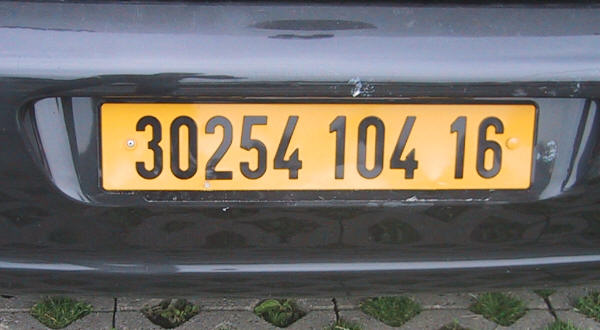Current passenger series since 1976, rear plate in black on yellow.
The registration consists of five numerals, followed by three numerals and two numerals.
The first numeral of the second group denotes the vehicle class where 1 stands for private cars.
The second and third numeral of that second group denote the year of manufacture where 04 stands for 2004.
The last two numerals denote the wilaya (state) where 16 stands for El Djezair (Algiers).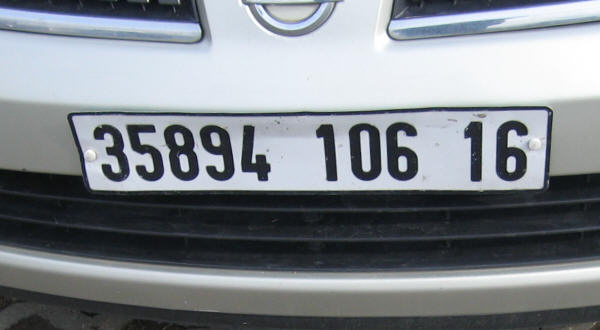Current passenger series since 1976, front plate in black on white.
The registration consists of five numerals, followed by three numerals and two numerals.
The first numeral of the second group denotes the vehicle class where 1 stands for private cars.
The second and third numeral of that second group denote the year of manufacture where 06 stands for 2006.
The last two numerals denote the wilaya (state) where 16 stands for El Djezair (Algiers).Current passenger series since 1976, rear plate in black on yellow.
The registration consists of five numerals above three numerals and two numerals.
The first numeral of the second group denotes the vehicle class where 1 stands for private cars.
The second and third numeral of that second group denote the year of manufacture where 06 stands for 2006.
The last two numerals denote the wilaya (state) where 16 stands for El Djezair (Algiers).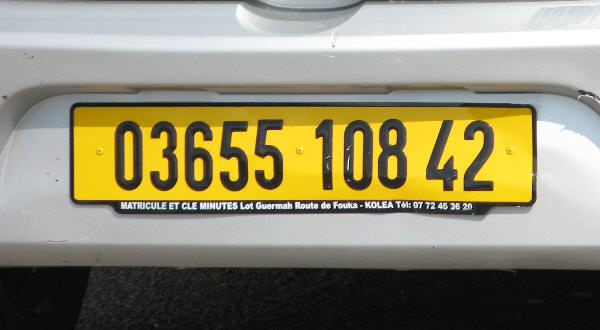Current passenger series since 1976, rear plate in black on yellow.
The registration consists of five numerals, followed by three numerals and two numerals.
The first numeral of the second group denotes the vehicle class where 1 stands for private cars.
The second and third numeral of that second group denote the year of manufacture where 08 stands for 2008.
The last two numerals denote the wilaya (state) where 42 stands for Tipaza.

## Diplomatic series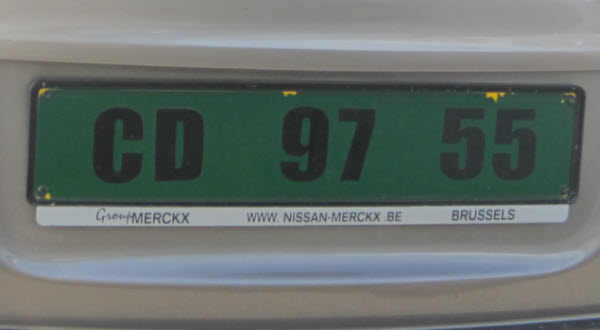Current diplomatic series in black on green.
The registration consists of the letters CD, followed by two numerals and two numerals.
The first two numerals denote the embassy where 97 stands for EU.Current diplomatic series in black on green.
The registration consists of the letters CMD, followed by two numerals and one numeral.
The letters CMD are used for the Ambassador.
The first two numerals denote the embassy where 97 stands for EU.Diplomatic series, front plate in black on white.
The registration consists of four numerals, followed by 66, three numerals and another two numerals.
66 stands for Diplomatic corps, 004 stands for 2004 and 23 denotes the state (wilaya) Annaba.
To the left of the plate is a blue band with the oval DZ in white above the twelve white European stars.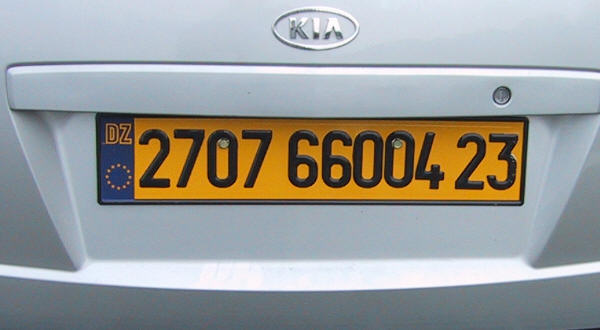Diplomatic series, rear plate in black on yellow.
The registration consists of four numerals, followed by 66, three numerals and another two numerals.
66 stands for Diplomatic corps, 004 stands for 2004 and 23 denotes the state (wilaya) Annaba.
To the left of the plate is a blue band with the oval DZ in yellow above the twelve golden European stars.